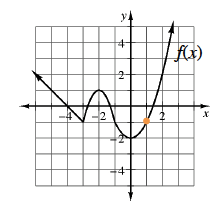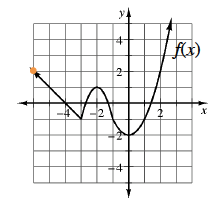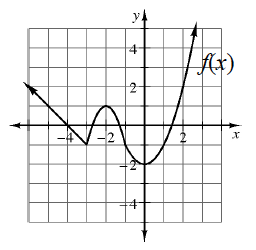### Home > GB8I > Chapter 8 Unit 9 > Lesson INT1: 8.1.3 > Problem8-51

8-51.

Use the graph of $f(x)$ at right to determine the following values.

1. $f(1)$

Find the point on the function that has an $x$-value equal to the input. Its y-value will equal the output.$f(1) = −1$

1. $f(−6)$1. $f(0)$

1. $f(−3)$